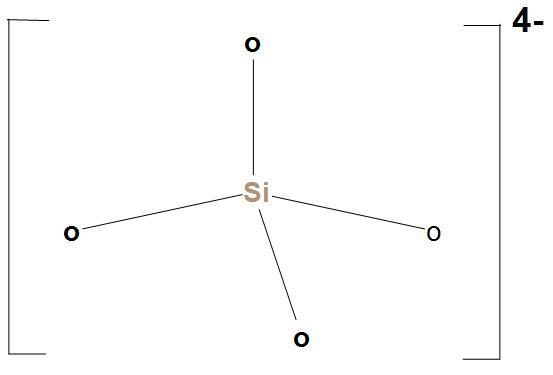QuestionAnswers

# The basic structural unit of silicates is:A. $Si{{O}^{-}}$B. $Si{{O}_{4}}^{4-}$C. $Si{{O}_{3}}^{2-}$D. $Si{{O}_{2}}^{2-}$

Hint: Silicate is actually a member of anions consisting of silicon and oxygen. It is found that these are stable and are fairly soluble in water. They also form several solid hydrates when crystallised from solution.
- $Si{{O}_{4}}^{4-}$ is called orthosilicate, which is the anion, or we can say any of its esters or salts. $Si{{O}_{4}}^{4-}$ is the basic structural unit of silicates. The structure of $Si{{O}_{4}}^{4-}$ is:Hence, we can say that the correct option is (B), that is the basic structural unit of silicates is $Si{{O}_{4}}^{4-}$.
Note: $Si{{O}_{4}}^{4-}$ is also called silicon tetroxide anion. We should not get confused in terms of silicon and silicone. The main difference between both of these is that Silicon is a naturally occurring chemical element. Whereas, silicone is a synthetic substance.